# Physics Assignment Help With Constraint Relations## How to determine Constraint Relation

1. Assume the direction of acceleration of each block, e.g. a1 (downward) and a2 (upward) in this case.

2. Locate the position of each block from a fixed point (depending on convenience), e.g. centre of the pulley in this case.

3. Identify the constraint and write down the equation of constraint in terms of the distance assumed.For example, in the chosen problem, the length of string remains constant is the constraint or restriction.
Thus,x1 + x2 = constant
Differentiating both the sides w.r.t. time, we get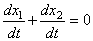Each term on the left side represents velocity of the block.
Since we have to find a relation between accelerations, therefore, we differentiate it once again w.r.t. time.
Thus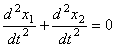Since the block m1 is assumed to be moving downward (x1 is increasing with time)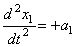, a1 > 0

and block m2 is assumed to be moving upward (x2 is decreasing with time)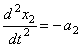, a2 > 0

Thus a1 - a2 = 0
or a1 = a2 = a

Alternatively, one can also assume the distance of each block from the ground as shown in the figure.
One can easily see that an expression for length of the string can not be written unless we locate the centre of the pulley w.r.t. ground.

If y0 be the distance of the ground from the centre of the pulley then the length of string is
(y0 -y1) + (y0 - y2) = constant
2y0 - y1 - y2 = constant

Differentiating twice w.r.t. time, we get
0 -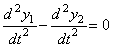Now,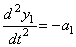and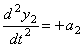Thus a1 - a2 = 0
a1 = a2 = aSubstituting a1 = a2 = a in equations (i) and (ii) and after solving them, we get
a =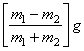also T =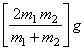### Homework Help For Constraint Relations

assignmenthelp.net provides best Online Assignment Help service in Physics for all standards. Our Tutor provide their high quality and optimized Tutorial help to fulfill all kind of need of Students.